# NCERT Solutions class 7 Maths Chapter-5 Exercise 5.2

NCERT Solutions Class-7 Maths chapter-5 line and angles Exercise-5.2 is prepared by academic team of pw all the questions of NCERT text book are solved step by step with proper and detail solutions explaining each and every questions . For More and additional questions of CBSE class 7 maths you can go to class 7 maths sections. NCERT class 7 Maths Solutions is the best way to enhanced your mathematics skill. And pw practice worksheet & question bank will help you a lot .

## NCERT Solutions class 7 Maths Chapter-5 line and angles

### Solutions of Chapter line and angles Exercise-5.2

Question 1:
State the property that is used in each of the following statements?
(i) If a||b, then ∠1 = ∠5
(ii) If ∠4 = ∠6, then a||b
(iii) If ∠4 + ∠5 = 180°, then a||b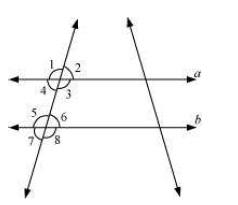(i) Corresponding angles property
(ii) Alternate interior angles property
(iii) Interior angles on the same side of transversal are supplementary.

Question 2:
(i) The pairs of corresponding angles
(ii) The pairs of alternate interior angles
(iii) The pairs of interior angles on the same side of the transversal
(iv) The vertically opposite angles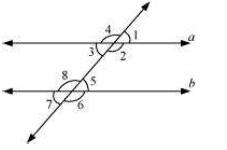(i) ∠1 and ∠5, ∠2 and ∠6, ∠3 and ∠7, ∠4 and ∠8
(ii) ∠2 and ∠8, ∠3 and ∠5
(iii) ∠2 and ∠5, ∠3 and ∠8
(iv) ∠1 and ∠3, ∠2 and ∠4, ∠5 and ∠7, ∠6 and ∠8

Question 3:
In the adjoining figure, p || q. Find the unknown angles.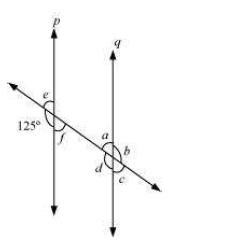∠d = 125° (Corresponding angles)
∠e = 180° − 125° = 55° (Linear pair)
∠f = ∠e = 55° (Vertically opposite angles)
∠c = ∠f = 55° (Corresponding angles)
∠a = ∠e = 55° (Corresponding angles)
∠b = ∠d = 125° (Vertically opposite angles)

Question 4:
Find the value of x in each of the following figures if l || m.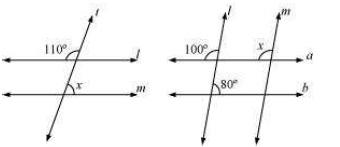(i)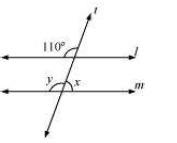∠y = 110° (Corresponding angles)
∠x + ∠y = 180° (Linear pair)
∠y = 180° − 110°
= 70°
(ii)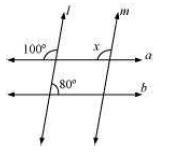∠x = 100° (Corresponding angles)

Question 5:
In the given figure, the arms of two angles are parallel.
If ∠ABC = 70°, then find
(i) ∠DGC
(ii) ∠DEF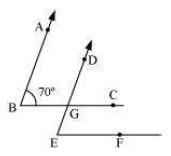(i) Consider that AB|| DG and a transversal line BC is intersecting them.
∠DGC = ∠ABC (Corresponding angles)
∠DGC = 70°
(ii) Consider that BC|| EF and a transversal line DE is intersecting them.
∠DEF = ∠DGC (Corresponding angles)
∠DEF = 70°

Question 6:
In the given figures below, decide whether l is parallel to m.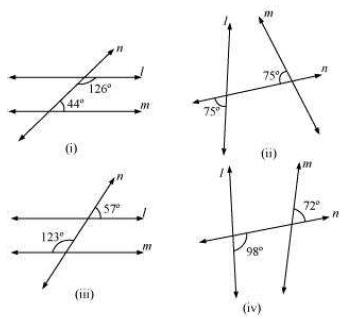(i)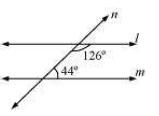Consider two lines, l and m, and a transversal line n which is intersecting them. Sum of the interior angles on the same side of transversal = 126º + 44º = 170° As the sum of interior angles on the same side of transversal is not 180º, therefore, l is not parallel to m.
(ii)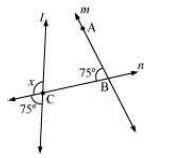x + 75° = 180° (Linear pair on line l)
x = 180° − 75° = 105°
For l and m to be parallel to each other, corresponding angles (∠ABC and ∠x)should be equal. However, here their measures are 75º and 105º respectively. Hence, these lines are not parallel to each other.
(iii)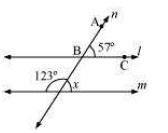∠x + 123° = 180° (Linear pair)
∠x = 180° − 123º = 57°
For l and m to be parallel to each other, corresponding angles (∠ABC and ∠x)should be equal. Here, their measures are 57º and 57º respectively. Hence, these lines are parallel to each other.
iv.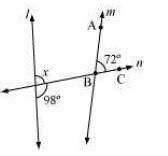98 + ∠x = 180° (Linear pair)
∠x = 82°
For l and m to be parallel to each other, corresponding angles (∠ABC and ∠x)should be equal. However, here their measures are 72º and 82º respectively. Hence, these lines are not parallel to each other.

NCERT CLASS 7 MATHEMATICS SOLUTIONS